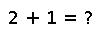# hash

hash(x[, h::UInt])

Compute an integer hash code such that `isequal(x,y)` implies `hash(x)==hash(y)`. The optional second argument `h` is a hash code to be mixed with the result.

New types should implement the 2-argument form, typically by calling the 2-argument `hash` method recursively in order to mix hashes of the contents with each other (and with `h`). Typically, any type that implements `hash` should also implement its own `==` (hence `isequal`) to guarantee the property mentioned above.

## Examples

Checking you are not a robot: